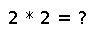# sortperm

``````..  sortperm(v, [alg=<algorithm>,] [by=<transform>,] [lt=<comparison>,] [rev=false])

Return a permutation vector of indices of ``v`` that puts it in sorted order.
Specify ``alg`` to choose a particular sorting algorithm (see Sorting Algorithms).
``MergeSort`` is used by default, and since it is stable, the resulting permutation
will be the lexicographically first one that puts the input array into sorted order –
i.e. indices of equal elements appear in ascending order. If you choose a non-stable
sorting algorithm such as ``QuickSort``, a different permutation that puts the array
into order may be returned. The order is specified using the same keywords as ``sort!``.

## Examples

Checking you are not a robot: